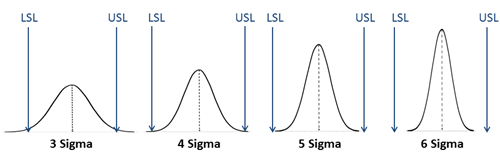# Don't Understand Cpk vs Ppk or Cp vs Pp?

## QI Macros Excel add-in will calculate them all for you.

### Cp and Cpk Process Capability Metrics

Cp is the capability index.
It measures how well the data might fit between the spec limits (USL, LSL). It doesn't care if the process is centered within the limits only if it would fit if it was centered.

Cpk is the centering capability index.
It measures how well the data is centered between the spec limits.

Use Cp Cpk  when you have a sample, not the population, and are testing the potential capability of a process to meet customer needs.

Cp and Cpk use Sigma estimator.

### Pp and Ppk Process Performance Metrics

Pp is the performance index.
It measures how well the data might fit between the spec limits (USL, LSL). It doesn't care if the process is centered within the limits only if it would fit if it was centered.

Ppk is the performance centering index.
It measures how well the data is centered between the spec limits.

Use Pp Ppk when you have the total population and are testing the performance of a system to meet customer needs.

Pp, Ppk use standard deviation.

### How do Cp and Cpk Relate to Sigma?

Cp Cpk
1.0
1.33
1.66
2.0
Sigma
3 Sigma
4 Sigma
5 Sigma
6 Sigma
% of Spec Tolerance Used
100%
75%
60%
50%### QI Macros Tools that Calculate Cp, Cpk, Pp, Ppk and Sigma

• Histogram - calculates Cp, Cpk, Pp, Ppk, Sigma and twenty other metrics using your data and spec limits.
• Capability Suite - creates six charts including histogram, control charts, probability plot, values plot and capability plot. Also calculates Cp, Cpk and Pp, Ppk.
• Cp Cpk Template - calculates Cp, Cpk and Pp, Ppk on multiple sets of data.